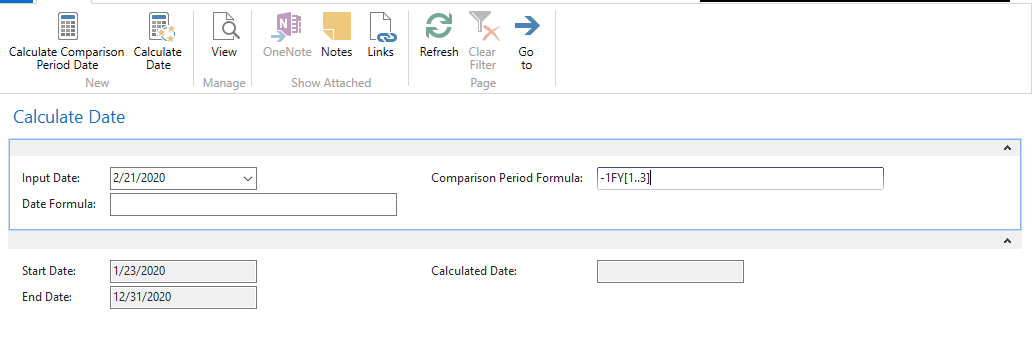#### Howdy, Stranger!

It looks like you're new here. If you want to get involved, click one of these buttons!

# Solved--Easy way to display Comparison Period Date?

Hello,

There is table 334 Column Layout and there are two fields there called "Comparison Period Formula" and "Comparison Date Formula".

What I would like to achieve is whatever formula I type in, I would like to see the date or date-range displayed.
What I have done so far is I created a page and was able to display calcdate formula result but I can't display the value I type into Comparison Period Formula.

For example, my input date is 02/21/2020
Comparison Period Formula: FY
I want to see what that range is.
I can pull up fy start date and fy end date from codeunit 8 account schedule management but I also want to use the Comparison Period Formula field.

I used FindEndOfFiscalYear and AccPeriodStartEnd functions to get FY start and end dates. Also tried to use AccPeriodStartEnd function to display calculated Comparison Period Formula but it doesn't display anything.

Calculate Date Action Code
```OutputDate := CALCDATE(DateFormula,InputDate);

CLEAR(InputDate);
CLEAR(DateFormula);
```

Calculate Comparison Period Date Code
```StartDate := AccSchedManagement.FindFiscalYear(InputDate);
EndDate := FindEndOfFiscalYear(InputDate);

//OutputDate := AccPeriodStartEnd(ComparisonPeriodFormula,InputDate,StartDate,EndDate);

CLEAR(InputDate);
CLEAR(ComparisonPeriodFormula);
```

Thank you in advance. This is a learning experience for me to understand Comparison Period Formula